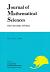## Vol. 3 (1996) No. 02Journal of Mathematical Sciences The University of Tokyo

1. Nakano, Fumihiko
Asymptotics of Heavy Molecules in High Magnetic Fields
Vol. 3 (1996), No. 2, Page 247--270.

2. Dzhuraev, Abduhamid
On Linear Boundary Value Problems in the Unit Ball of $\Bbb C^n$
Vol. 3 (1996), No. 2, Page 271--295.

3. Miyazaki, Takuya
Mixed Hodge Structures of Siegel Modular Varieties and Siegel Eisenstein Series
Vol. 3 (1996), No. 2, Page 297--330.

4. Taniguchi, Kenji
Discrete Series Whittaker Functions of $SU(n, 1)$ and $Spin(2n, 1)$
Vol. 3 (1996), No. 2, Page 331--377.

5. Hattori, Kumiko
Fractal Geometry of Self-avoiding Processes
Vol. 3 (1996), No. 2, Page 379--397.

6. Oka, Mutsuo
Two Transforms of Plane Curves and Their Fundamental Groups
Vol. 3 (1996), No. 2, Page 399--443.

7. Markushevich, Dimitri
Minimal Discrepancy for a Terminal cDV Singularity Is 1
Vol. 3 (1996), No. 2, Page 445--456.
On the Iwasawa λ-invariant of the Real $p$-cyclotomic Field
Isometries on the $\ell^p$-sum of $C_0(Î©, E)$ Type Spaces